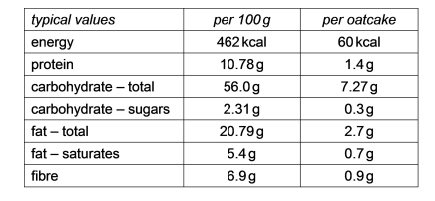# IMAT 2018 Q17 [Oatcakes]

A 300 g packet of Scottish oatcakes has the following information on the side of the packet:How many oatcakes are there in the packet?

A. 23
B. 24
C. 18
D. 29
E. 21

1 Like

Simple steps to solve word problems:

• Underline key information

• Determine what they are trying to ask, and what you will need to solve it

• Eliminate any non-essential information

• Draw a picture, graph, or equation

• In moments of high stress like exam taking, always work with the paper they give you to avoid careless mistakes.

• Solve.

With a calculator, this question would be a piece of cake, but since we are missing one for this exam, we need to do some estimation. Instead of being overwhelmed with the complicated table of numbers, pick one value and then find out how much of it would be in the pack. After we have this, we can figure out how much would be per cake.

Fibre is the easiest number to try because 6.9g is close to 7g and 0.9g is very close to 1g, so it makes the math a bit easier.

Per 100g we have 5.9g, so per pack (3)(100g) = 300g, we must have (3)(6.9g) = 20.7g

Now we know the total fibre content, we can find out how many oatcakes there are per pack.

Let’s break the numbers down very simply at first. Start with guessing if 10 cakes could fit in the pack.

(20.7g) - (10)(0.9g) = 11.7g of fiber left. We can clearly have 20 cakes as this is more than double what we just subtract.

(20.7g) - (20)(0.9g) = 20.7g - 18.0g = 2.7g

Now you can just subtract 2.7g - 0.9g over and over again if you are not sure, but it should be easy to see that 2,7g/0.9g = 3

So let’s add up all the cakes we just calculated: total cakes = 20 + 3 = 23

\fcolorbox{red}{grey!30}{Therefore the answer must be A, \$23\$..}

Notice how, even though the numbers seem complicated, they work out perfectly in the end? On the exam, you should not be afraid to round, but when the answers are as close as they are in this case (18, 21, 23, 24), you need to be careful and not round unless absolutely necessary.